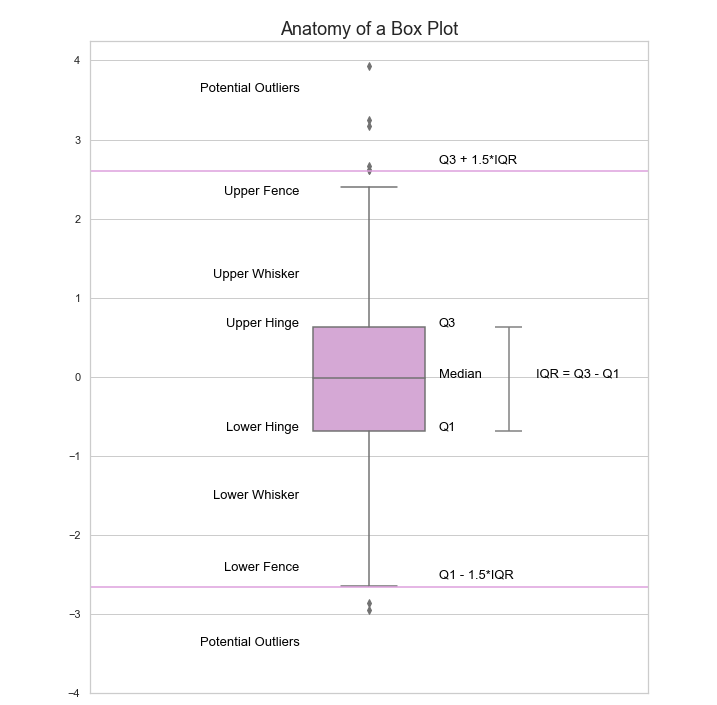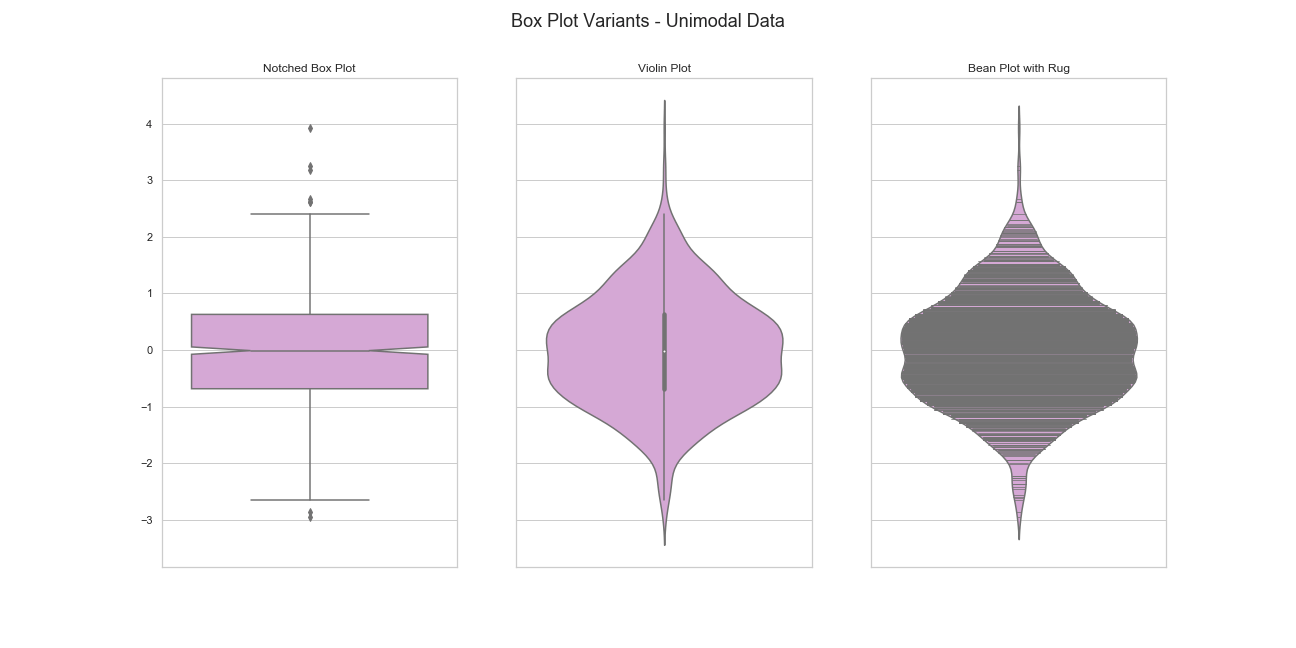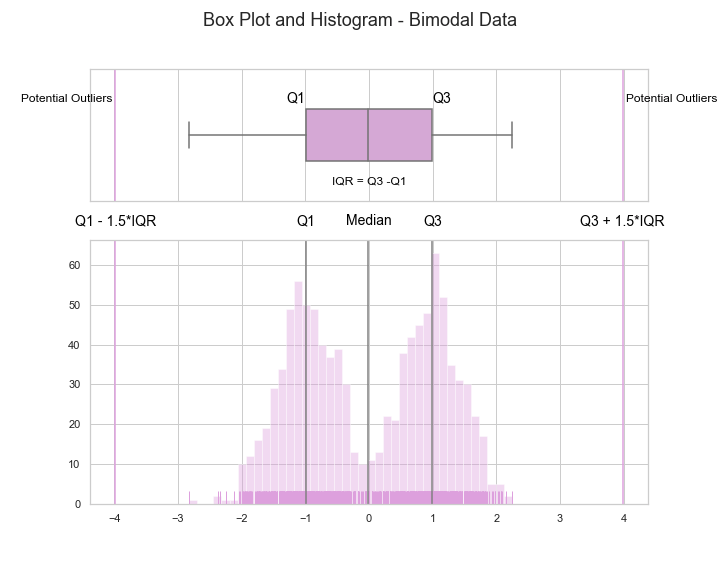# Anatomy of a Box Plot

This post is dedicated to one of the most popular tools in data visualisation: the Box Plot, a simple tool which was introduced 40 years ago but remains in fashion.

Box plots, also called  Box and whisker plots, are one of the most frequently used graphs to visualise data. They were introduced by the American statistician John Tukey around 1970 and became widely known after the publication of his book Exploratory Data Analysis in 1977 (yes, you can buy it on Amazon!).

Nowadays, more than 40 years after their official introduction,  Box plots are still widely used in academia as well as across all kinds of industries. They have demonstrated to be useful for revealing the central tendency and variability of the data, the distribution (symmetry or skewness) shape, and the possible presence of outliers. Moreover, they are also a powerful graphical technique for comparing samples from two or more different populations (as we observed in previous post Comparison of Two Populations).

Box Plots are made of five key components which together allows to get some information about the distribution of our data:

• Median
• Hinges: two hinges located at the lower and the upper quartiles denoted by Q1 and Q3, respectively
• Fences: two fences determined as the data values which are adjacent to the extremes:

Lower Extreme = Q1 – 1.5(IQR),

Upper  Extreme = Q3 + 1.5(IQR),

where IQR denotes the inter quartile range (IQR = Q3 – Q1).

• Whiskers: two lines that connect the hinges with the fences
• Potential Outliers: all individual points further away from the lower and upper extremes are represented as dots.

These elements are illustrated in the following figure.Box Plot key components as proposed by John Tukey in 1977.

In this example we can observe that 50% of the data points are contained in the region determined by the box (i.e. half of our data are in contained in an interval around zero). Besides,  the fact that the upper whisker does not reach the upper extreme shows that the biggest sample point within the extremes (upper fence) is strictly smaller than the upper extreme. Finally, we can see that there are some potential outliers in both sides of the distribution.

## Variations

Box plots have evolved and many variations have been proposed during the past 40 years. For example, Notched Box Plots were introduced by McGill R, Tukey, and Larsen in their paper Variations of Box Plots. This variation shows the number of observations in a batch using the width of the box, while the notches give an indication of the statistical difference between two batches.

Violin Plots were introduced by Jerry L. Hintze and Ray D. Nelson in their paper Violin Plots: A Box Plot-Density Trace Synergism in 1998. A violin plot consists of a density trace combined with the quartiles of a box plot. It is worth noting that individual outliers are not illustrated in a violin plot.

Last but not least, Bean Plots were introduced in  2008 by Peter Kampstra in his paper Beanplot: A Boxplot Alternative for Visual Comparison of Distributions. These provide a side-by-side display that contains the density curve, the original observations that generated the density curve in a rug-plot, and the mean of each group.Some of the variants of Box Plots that have been proposed over time.

## Combining Tools

As we mentioned, box plots are a great tool in exploratory data analysis. However, one should not rely on a single visualisation tool for analysing data. Instead, we should combine and compare different tools and techniques in order to be get to know our data set. A good starting point can be combining a Box Plot and a Histogram with a Rug (1d scatter plot). Take a the two examples below.

Example 1 (Unimodal Data). In this example we used a sample (size n = 1000) from a normal distribution with zero mean and variance one. As we can see below, the Box Plot does a pretty good job at summarising the distribution and conveys more o less the same information that the histogram. Besides, it help us to identify the presence of potential outliers in the sample so we can investigate further.Example 2 (Bimodal Data). In this example we used a sample (size n=1000) from a mixture of normal with two modes. In this case, the Box Plot provides useful information about the median, range, and inter quantile range but fails to illustrate the bimodal nature of the data which is well-captured by the histogram.Using a combination of graphical tools we can perform efficient exploratory analysis and get deeper insights about the distribution of our data.